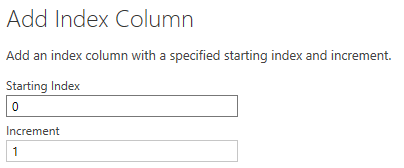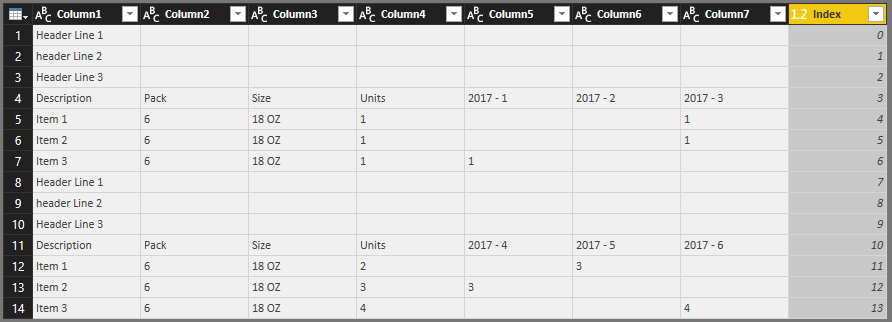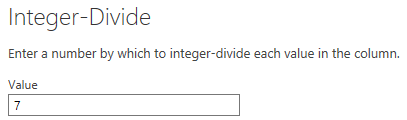I was answering a question on the Power BI Community Forum where a user was looking to get some data from an Excel spreadsheet into Power BI. And in order for me to complete it, I wanted to get the same row number for x number of rows.

Example

• I had some sample data where the same data was repeated every 7 rows
•• What I wanted is that for every 7 rows the Index number to be the same.

# How I did it

• What I did first was to create an Index Column, starting at 0
•• This is what the table looked like once completed
•• I then used the Standard Button on the Transform Ribbon and selected “Integer-Divide”
•• Now because I wanted this to be the same number for 7 rows at a time I put in 7
•• When then gave me the desired result, as you can see below, I have got 0 for the first 7 rows and 1 for the next 7 rows (8-14)
•